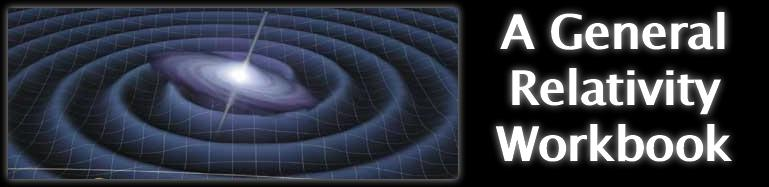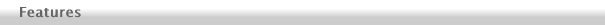This page summarizes and illustrates some of the most important features of A General Relativity Workbook. The links are to PDF files.Each chapter is designed to correspond to a single (50-minute) class session.Each chapter title page has a flow chart thatShows how different sections of the book are relatedShows how the current chapter fits into that structureEach chapter begins with a Concept Summary that provides an overview of the important concepts without derivations or other distractions. Here is an example Concept Summary page.The Concept Summary is linked to Boxes which discuss details omitted from the summary and encourage students to work through derivations and/or practice using the ideas discussed. Each box provides room on the page for student work. Here is an example box page.Homework problems at the end of each chapter provide more opportunities for student practice and class discussion. Here is an example homework problems page.Physics, physics applications, and intuitive insight are emphasized over the math. The mathematical formalism is developed only to the level really required to understand the physics.The math is developed slowly, giving students plenty of time to become familiar with basic aspects of the formalism before moving on to more complex aspects. Students are also given plenty of practice and guidance in avoiding common problems with the notation. A Diagonal Metric Worksheet is used to help students quickly and accurately evaluate Christoffel symbols and Ricci tensor components. This provides a low-tech alternative to tedious direct calculations or expensive and hard-to-use symbolic math computer applications. Here is the worksheet.The book uses computer models to explore applications (such as the time-evolution of our actual universe) that cannot be treated analytically. Moreover, the book teaches (and has students practice) computer modeling techniques to that these applications are not mysterious black boxes. See the resources page for some examples.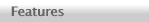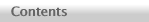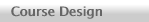You can purchase a copy directly from the publisher here.New! Online Student Manual available here.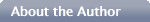Thomas A. Moore has been a professor in the Physics and Astronomy Department at Pomona College since 1987. He does theoretical research on detecting gravitational waves using LISA (now eLISA). Send him a message.Copyright © 2009, 2010, 2015 Thomas A. Moore